# Current Amplifier for Arbitrary Waveform Generator

1
3917

Hi,

Another quick post before whilst we get our ChipKit tutorial finished today; we have been designing a circuit which is powered from a thermal battery. These have a fairly slow voltage ramp rate when they are first powered on, and so circuits need to be tested to make sure they work with this slow increase in voltage.
Repeatedly doing this with batteries gets expensive rapidly and we had a capture on the oscilloscope which describes how the voltage increases. We can save this capture as a comma separated values (CSV) file. We can output this CSV file from an arbitary waveform generator, so the obvious next step was to amplify the signal (increase the current) so we could run the circuitry from a power supply.

The easiest way to do this is to use a common collector circuit. This is a single transistor amplifier that can provide more current than the input (current gain), and outputs the same voltage-exactly what we want!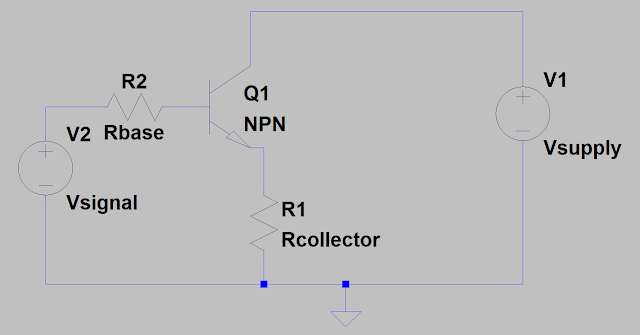So lets pick some basic components and simulate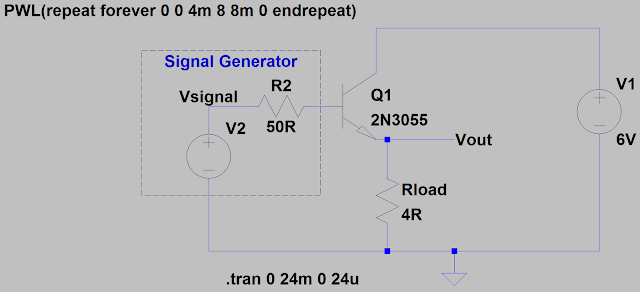We’ll use a triangle wave input (in green), the output is in blue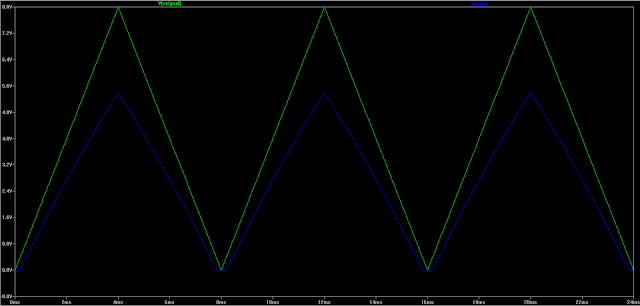The output looks basically good, but there is a voltage drop as well as some distortion at the lower end. We can re-run the simulation with a lower voltage input to see this distortion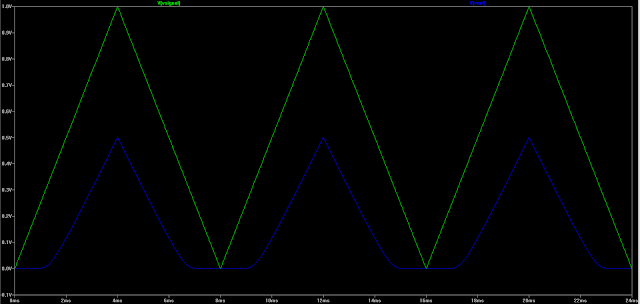As you can see, it’s not behaving itself. Let’s plot Vin vs Vout to see what’s happening.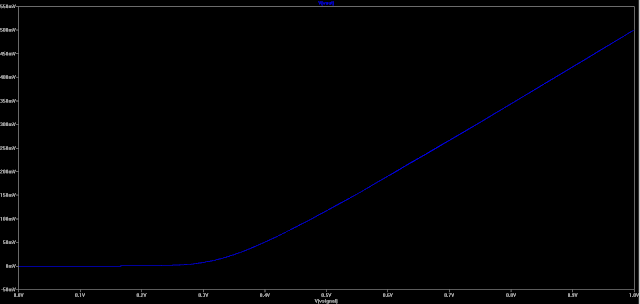It isn’t working as a (linear) current amplifier below 0.4V. This is because the transistor requires a certain voltage between the base and emitter in order for it to start turning on. For most applications, we can use a common potential divider biasing circuit as shown below.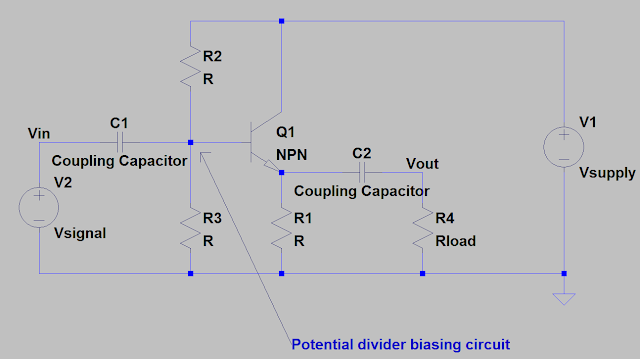However, this won’t work in this case as this common biasing circuit is AC coupled, and this amplifier needs to work for signals down to DC. What we can do to solve the distortion and biasing is to use negative feedback. Basically we look at what the actual output is and compare this with what we would like the output to be. The fastest way to implement this is the old favourite, the operational amplifier.

So lets put an op-amp in the circuit. This will handle biasing the transistor, as well as compensating for any non-linearity in the circuit. So the circuit will now look like,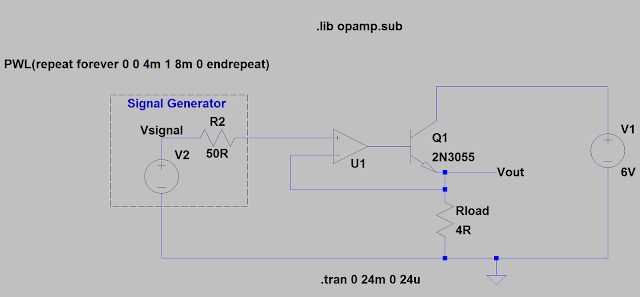So, let’s simulate with the same triangle wave as we had before (using an ideal op-amp)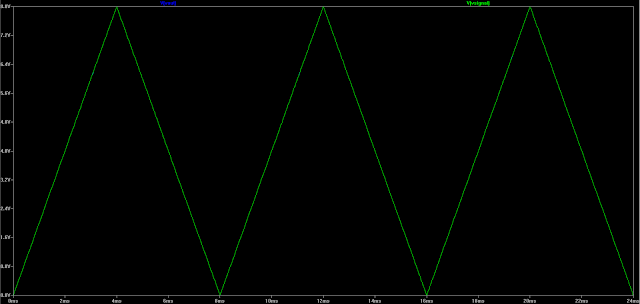And the Vin vs Vout plot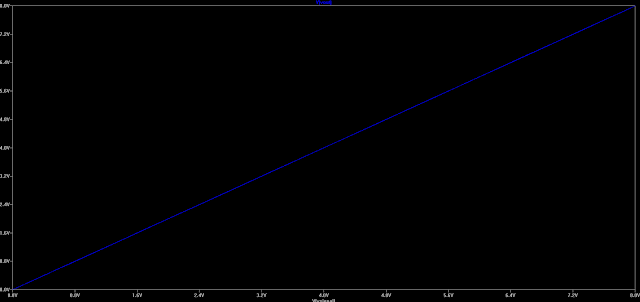As you can see, the output now follows the input precisely (they are both on there, just overlaid!), and if we try it again with a different load (80ohms), the circuit should still amplify nicely.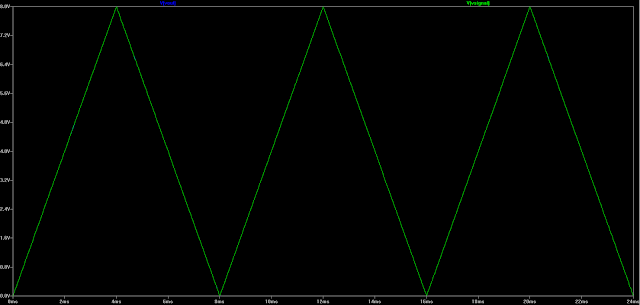Looks like it’s working, lets build it! The op-amp selection isn’t critical for this particular application as it isn’t running at a particularly high speed. The op-amp used was a MC4558CN. This has an 5.5MHz gain bandwidth product so this shall be more than fast enough for the application. It will be running off a single supply, but we don’t need to have an output near the power supply rails (0V & 8V), so we don’t need to use a rail to rail output amplifier.

Selecting the output transistor is also not critical. We are looking for an NPN transistor with a reasonable current gain. Also, as we are using this as a linear amplifier (class B amplifier, or one half of a push-pull output stage), we are expecting the transistor to dissapate a fairly large amount of power. A safe choice, and more importantly, one that was in stock, was the classic 2N3055 transistor. This has a transistion frequency of 3MHz, so we know at this point that our current gain will drop to 1. However, we are operating far below this frequency. Importantly, as this is in a TO-3 case, we can dissipate up to 115W of power into this transistor, assuming it is properly heatsinked.

A quick build on veroboard later,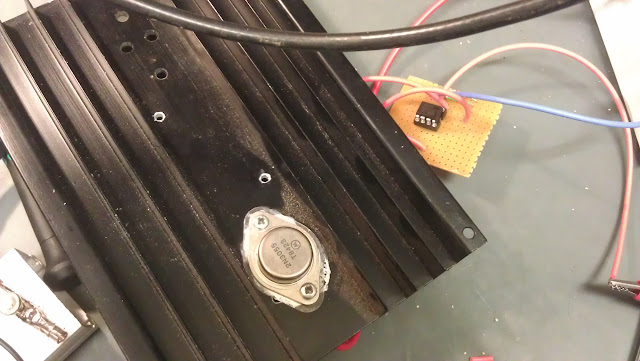The performance is pretty good. I put in a sin wave from the function generator and the output tracked the input really well.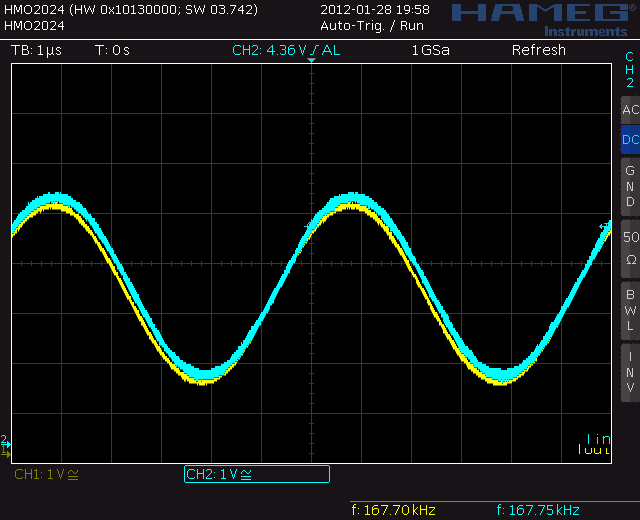It was tried with a 500 ohm load and a 4 ohm power resistor-performance was identical. But what about frequency performance?

If we take the freqency up to about 220kHz, then we start to get some distortion at the output.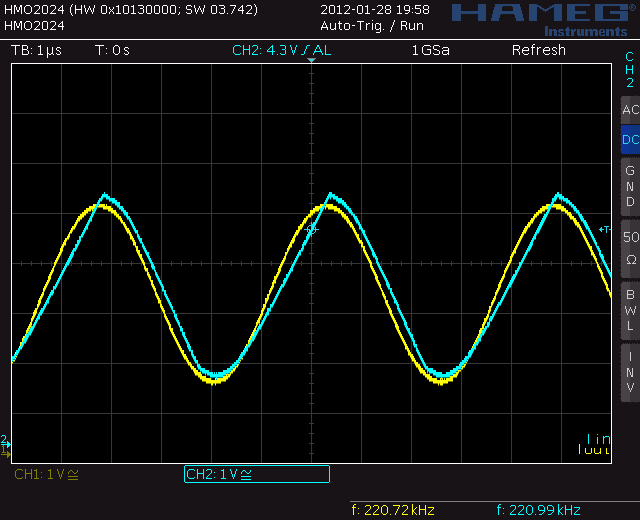Looking at a lissajous curve, we get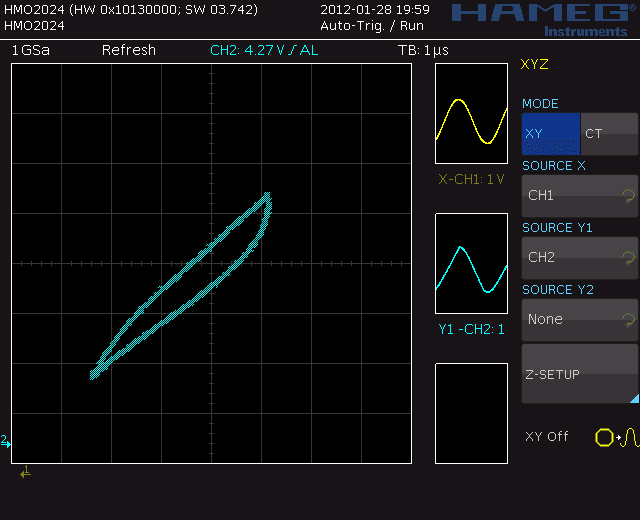Below this frequency it works pretty nicely. For the last test, we have a look at the response to a step change in load. In this case I went from a 1k load to a 22R load and scoped the result. We could improve this with some capacitive terms in the feedback, but not too bad for a 20minute lash up job!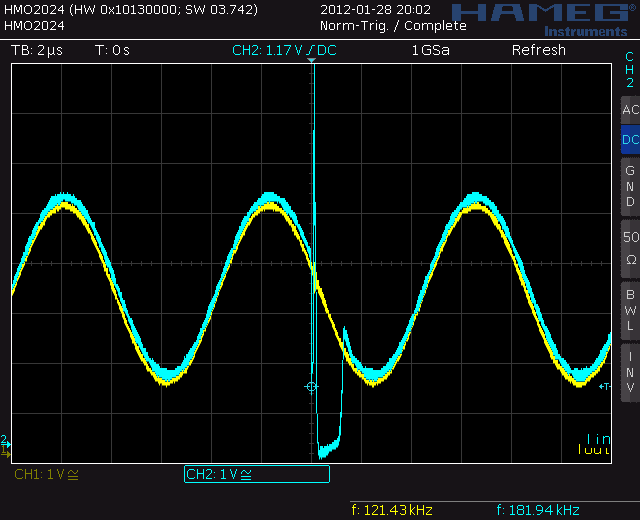#### 1 COMMENT

1. choosing frequency spec alone from opamp is not enough for this kind of large signal high frequency circuit. one other factor need to be considered is slew rate. i had to choose an opamp thats overkill by frequency spec alone, something like 350MHz unity gain BW to be worked in only 1-10MHz system (at unity gain), this is because this opamp only in my stock thats capable to cope with V/us (slew rate) specification from my circuit. even the 4558 used has well over the frequency capability, but slew rate formula SL = 2pi*f*Vpeak will limit the frequency capability based on required Vpeak and SL opamp spec to avoid distortion. YMMV.